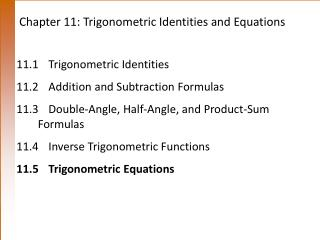DownloadDownload PresentationChapter 11: Trigonometric Identities and Equations

# Chapter 11: Trigonometric Identities and Equations

Download Presentation## Chapter 11: Trigonometric Identities and Equations

- - - - - - - - - - - - - - - - - - - - - - - - - - - E N D - - - - - - - - - - - - - - - - - - - - - - - - - - -
##### Presentation Transcript

1. Chapter 11: Trigonometric Identities and Equations 11.1 Trigonometric Identities 11.2 Addition and Subtraction Formulas 11.3 Double-Angle, Half-Angle, and Product-Sum Formulas 11.4 Inverse Trigonometric Functions 11.5 Trigonometric Equations

2. 11.5 Trigonometric Equations Solving a Trigonometric Equation by Linear Methods Example Solve 2 sin x– 1 = 0 over the interval [0, 2). Analytic Solution Since this equation involves the first power of sin x, it is linear in sin x. Two x values that satisfy sin x = ½ for are However, if we do not restrict the domain there will be an infinite amount of answers since: for k any integer.

3. 11.5 Solving a Trigonometric Equation by Linear Methods Graphing Calculator Solution Graph y = 2 sin x– 1 over the interval [0, 2]. The x-intercepts have the same decimal approximations as

4. 11.5 Equations Solvable by Factoring Example Solve 2sin2x– sin x – 1 = 0 Solution This equation is of quadratic form so: The solutions for sinx = - ½ in [0, 2) are x= The solutions for sin x = 1 in [0, 2) is x = Thus the solutions are:

5. 11.5 Solving a Trigonometric Equation by Factoring Example Solve sin x tan x = sin x. Solution Caution Avoid dividing both sides by sin x. The two solutions that make sin x = 0 would not appear.

6. 11.5 Solving a Trigonometric Equation by Squaring and Trigonometric Substitution Example Solve over the interval [0, 2). Solution Square both sides and use the identity 1 + tan2x = sec2x. Possible solutions are: Or are they? Check answers!

7. 11.5 Trigonometric Equations Solving an Equation Using a Double-Number Identity Example Solve cos 2x = cosx over the interval [0, 2). Analytic Solution Solving each equation yields the solution set

8. 11.5 Solving an Equation Using a Double- Number Identity Graphical Solution Graph y = cos 2x–cosx in an appropriate window, and find the x-intercepts. The x-intercept displayed is 2.0943951, an approximation for 2/3. The other two correspond to 0 and 4/3.

9. 11.5 Solving an Equation Using a Double-Number Identity Example Solve 4 sin xcosx = over the interval [0, 2). Solution From the given domain for x, 0  x < 2, the domain for 2x is 0  2x < 4. Since 2 sin xcosx = sin 2x.

10. 11.5 Solving an Equation that Involves Squaring Both Sides Example Solve tan 3x + sec 3x = 2 Solution Since the tangent and secant functions are related by the identity 1 + tan2 = sec2 , we begin by expressing everything in terms of secant. Square both sides. Replace tan2 3x with sec2 3x – 1.

11. 11.5 Solving an Equation that Involves Squaring Both Sides